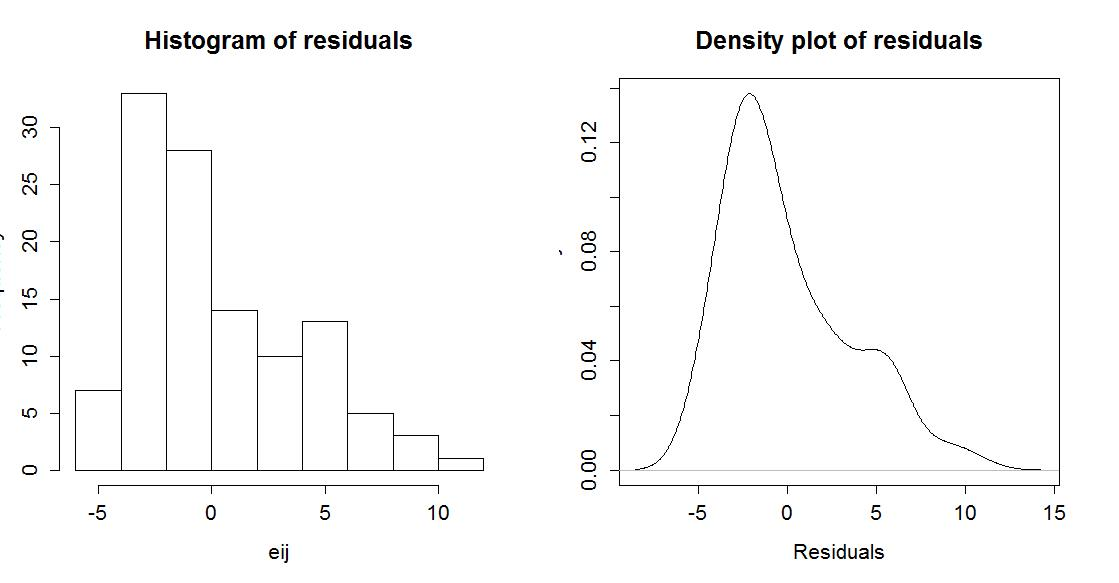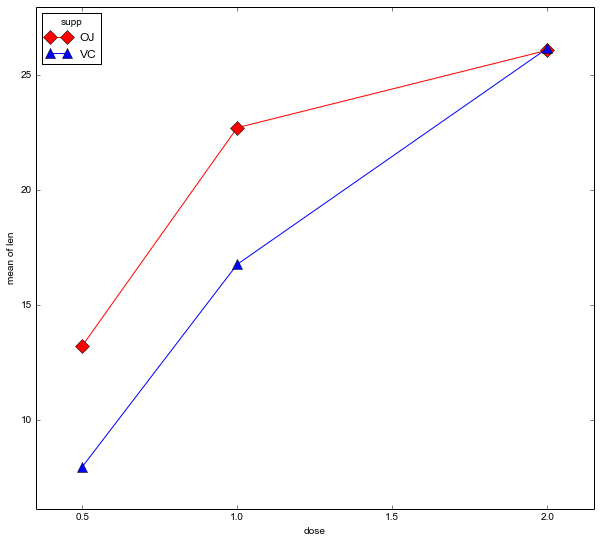# ANOVA, ANCOVA, MANOVA, & MANCOVA

Comparison Chart

 BASIS FOR COMPARISON ANOVA ANCOVA Meaning ANOVA is a process of examining the difference among the means of multiple groups of data for homogeneity. ANCOVA is a technique that remove the impact of one or more metric-scaled undesirable variable from dependent variable before undertaking research. Uses Both linear and non-linear model are used. Only linear model is used. Includes Categorical variable. Categorical and interval variable. Covariate Ignored Considered BG variation Attributes Between Group (BG) variation, to treatment. Divides Between Group (BG) variation, into treatment and covariate. WG variation Attributes Within Group (WG) variation, to individual differences. Divides Within Group (WG) variation, into individual differences and covariate.

## Analysis of variance (ANOVA)

ANOVA is a collection of statistical models and their associated procedures (such as “variation” among and between groups) used to analyze the differences among group means. In the ANOVA setting, the observed variance in a particular variable is partitioned into components attributable to different sources of variation. In its simplest form, ANOVA provides a statistical test of whether or not the means of several groups are equal, and therefore generalizes the t-test to more than two groups. ANOVA is useful for comparing (testing) three or more means (groups or variables) for statistical significance. It is conceptually similar to multiple two-sample t-tests, but is more conservative (results in less type I errorand is therefore suited to a wide range of practical problems.

## Assumptions for ANOVA

• Each group sample is drawn from a normally distributed population
• All populations have a common variance
• All samples are drawn independently of each other
• Within each sample, the observations are sampled randomly and independently of each other

After fitting an ANOVA model it is important to always check the relevant model

assumptions. This includes making QQ-plots and residual plots.Python code for 2-way ANOVA

 1 2 3 4 5 6 import pandas as pd from statsmodels.formula.api import ols from statsmodels.stats.anova import anova_lm from statsmodels.graphics.factorplots import interaction_plot import matplotlib.pyplot as plt from scipy import stats
datafile=“ToothGrowth.csv”
fig = interaction_plot(data.dose, data.supp, data.len,
colors=[‘red’,‘blue’], markers=[‘D’,‘^’], ms=10)ANOVA models:

1. Fixed-effects models which assume that data from normal populations that differ in their means allows the estimation of the range of response that any treatments towards them will generate.
2. Random-effects models which assume that data from a constrained hierarchy of different populations are sampled with different factor levels.
3. Mixed-effects models which describe the situations where both fixed and random effects are present.

Types of ANOVA:

One-way ANOVA, is used to test for differences among two or more independent groups.
Factorial ANOVA, is used in the study of the interaction effects among treatments.
Repeated measures ANOVA, is used when the same subject is used for each treatment.
Multivariate analysis of variance (MANOVA), is used when there is more than one response variable## Analysis of covariance (ANCOVA)Analysis of covariance (ANCOVA) combines features of both ANOVA and
regression. It augments the ANOVA model with one or more additional
quantitative variables, called covariates, which are related to the response
variable. The covariates are included to reduce the variance in the error terms
and provide more precise measurement of the treatment effects. ANCOVA is
used to test the main and interaction effects of the factors, while controlling for
the effects of the covariate.

ANCOVA is a regression with qualitative and continuous covariates, but without interaction terms between the factors and the continuous explanatory variables (i.e., the so called ‘parallel slopes assumption’).

ANCOVA can be used

(1) Adjusting preexisting differences in nonequivalent (intact) groups.

This controversial application aims at correcting for initial group differences (prior to group assignment) that exists on DV among several intact groups. In this situation, participants cannot be made equal through random assignment, so CVs are used to adjust scores and make participants more similar than without the CV. However, even with the use of covariates, there are no statistical techniques that can equate unequal groups. Furthermore, the CV may be so intimately related to the IV that removing the variance on the DV associated with the CV would remove considerable variance on the DV, rendering the results meaningless(2) Increase statistical power when one exists by reducing the within-group error variance.

In order to understand this, it is necessary to understand the test used to evaluate differences between groups, the F-test. The F-test is computed by dividing the explained variance between groups (e.g., gender difference) by the unexplained variance within the groups. Thus,## Assumptions of ANCOVA

There are several key assumptions that underlie the use of ANCOVA and affect interpretation of the results. The standard linear regression assumptions hold; further we assume that the slope of the covariate is equal across all treatment groups (homogeneity of regression slopes).

Assumption 1: linearity of regression

The regression relationship between the dependent variable and concomitant variables must be linear.

Assumption 2: homogeneity of error variances

The error is a random variable with conditional zero mean and equal variances for different treatment classes and observations.

Assumption 3: independence of error terms

The errors are uncorrelated. That is, the error covariance matrix is diagonal.

Assumption 4: normality of error terms

The residuals (error terms) should be normally distributed {\displaystyle \epsilon _{ij}}~ {\displaystyle N(0,\sigma ^{2})}.

Assumption 5: homogeneity of regression slopes

The slopes of the different regression lines should be equivalent, i.e., regression lines should be parallel among groups.

The fifth issue, concerning the homogeneity of different treatment regression slopes is particularly important in evaluating the appropriateness of ANCOVA model. Also note that we only need the error terms to be normally distributed. In fact both the independent variable and the concomitant variables will not be normally distributed in most cases.

Two-way Analysis of Variance
Two-way ANOVA is used to compare the means of populations that are
classified in two different ways, or the mean responses in an experiment with two
factors. We fit two-way ANOVA models in R using the function lm(). For
example, the command:
> lm(Response ~ FactorA + FactorB)
fits a two-way ANOVA model without interactions. In contrast, the command
> lm(Response ~ FactorA + FactorB + FactorA*FactorB)
includes an interaction term. Here both FactorA and FactorB are categorical
variables, while Response is quantitative.

Analysis of Covariance (ANCOVA)

Prior to performing ANCOVA it is sensible to make a scatter plot of the response
variable against the covariate, using separate symbols for each level of the
factor(s). This allows one to verify the assumptions that there is a linear
relationship between the covariate and the response variable, and that all
treatment regression lines have the same slope.

Ex. A company studied the effects of three different types of promotions on the
sales of a specific brand of crackers:
Treatment 1 – The crackers were on their regular shelf, but free samples
were given in the store,
Treatment 2 – The crackers were on their regular shelf, but were given
Treatment 3 – The crackers were given special display shelves at the end
of the aisle in addition to their regular shelf space.

The company selected 15 stores to participate in the study. Each store was
randomly assigned one of the 3 promotion types, with 5 stores assigned to each
promotion. Data was collected on the number of boxes of crackers sold during
the promotion period, y, as well as the number sold during the proceeding time
period, denoted x.
The following commands read the data:
> dat
Treatment Sale Presale
1 T1 38 21
2 T2 43 34
3 T3 24 23
4 T1 39 26
….
14 T2 34 25
15 T3 28 29
Prior to performing ANCOVA it is sensible to make a scatter plot of the response
variable against the covariate, using separate symbols for each level of the
factor(s). This allows one to verify the assumptions that there is a linear
relationship between the covariate and the response variable, and that all
treatment regression lines have the same slope.
> plot(Presale[Treatment == ‘T1’], Sale[Treatment == ‘T1′], xlab=’Presale’,
ylab=’Sale’, xlim=c(15,35), ylim=c(20,46), pch=15, col=’green’)
> points(Presale[Treatment == ‘T2’], Sale[Treatment == ‘T2′], pch=15, col=’red’)
> points(Presale[Treatment == ‘T3’], Sale[Treatment == ‘T3′], pch=15, col=’blue’)

As it appears that both the linearity and equal slopes assumptions required for
ANCOVA are valid we are able to proceed with the analysis. The following code
performs a one-way ANCOVA model, controlling for the sales in the proceeding
time period:
> results = lm(Sale ~ Presale + Treatment)
> anova(results)
Analysis of Variance Table
Response: Sale
Df Sum Sq Mean Sq F value Pr(>F)
Presale 1 190.68 190.678 54.379 1.405e-05 ***
Treatment 2 417.15 208.575 59.483 1.264e-06 ***
Residuals 11 38.57 3.506

Signif. codes: 0 ‘***’ 0.001 ‘**’ 0.01 ‘*’ 0.05 ‘.’ 0.1 ‘ ’ 1
The output tells us that the three cracker promotions differ in effectiveness (F =
59.48, p-value < 0.0001). One can now continue by using multiple comparison
techniques to determine how they differ. Note that we also need to check the
residuals to determine whether the other model assumptions hold.